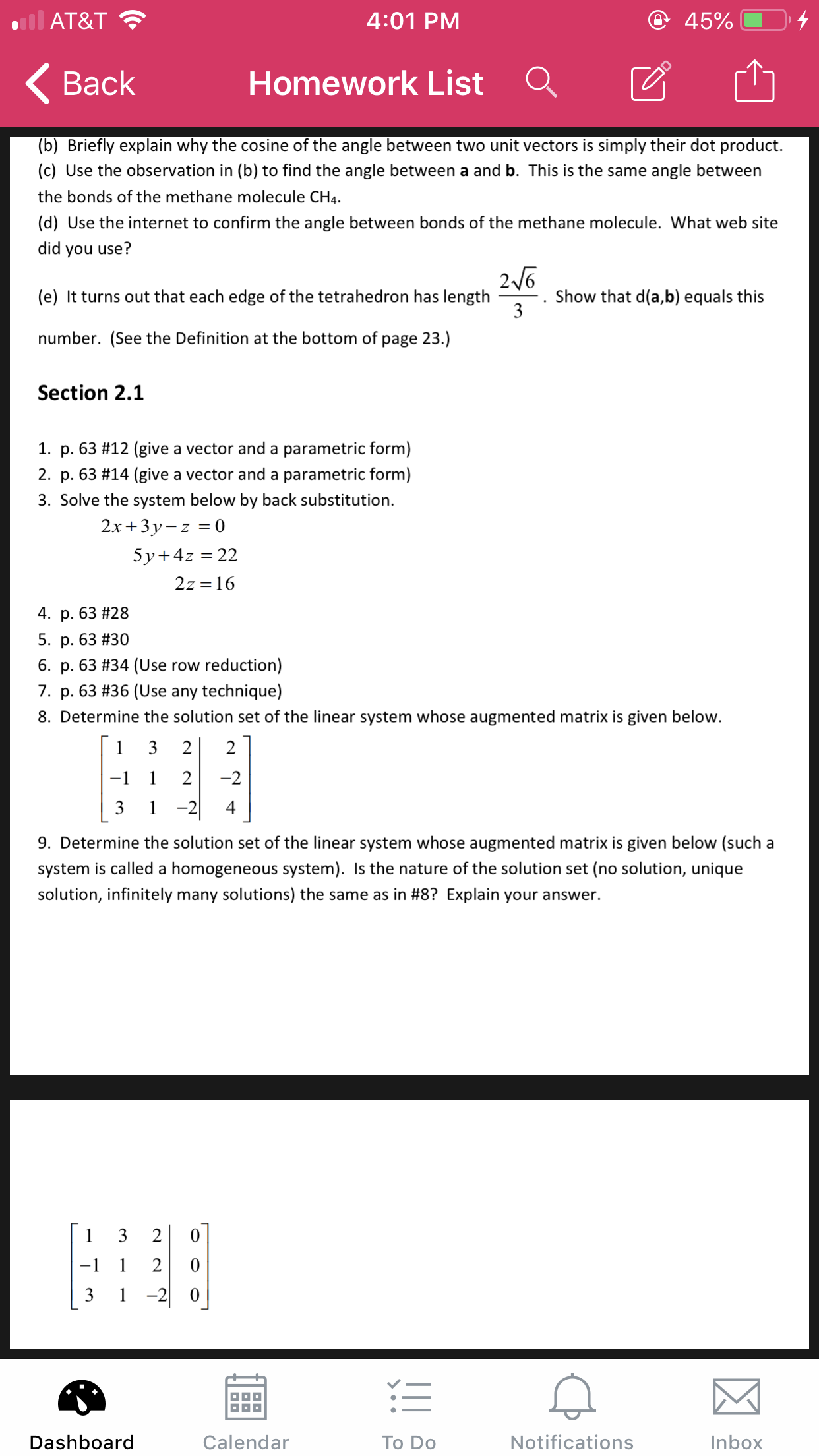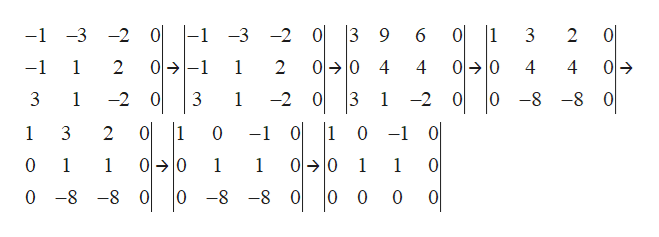# @ 45%AT&T4:01 PMHomework ListВack(b) Briefly explain why the cosine of the angle between two unit vectors is simply their dot product.(c) Use the observation in (b) to find the angle between a and b. This is the same angle betweenthe bonds of the methane molecule CH4(d) Use the internet to confirm the angle between bonds of the methane molecule. What web sitedid you use?26Show that d(a,b) equals this3(e) It turns out that each edge of the tetrahedron has lengthnumber. (See the Definition at the bottom of page 23.)Section 2.11. p. 63 #12 (give a vector and a parametric form)2. p. 63 #14 (give a vector and a parametric form)3. Solve the system below by back substitution2.x+3y-z05y 4z 222z 164. p. 63 # 285. p. 63 #306. p. 63 #34 (Use row reduction)7. p. 63 #36 (Use any technique)8. Determine the solution set of the linear system whose augmented matrix is given below.32212-2-1-2|3149. Determine the solution set of the linear system whose augmented matrix is given below (such asystem is called a homogeneous system). Is the nature of the solution set (no solution, uniquesolution, infinitely many solutions) the same as in #8? Explain your answer.132-1121-20NotificationsDashboardCalendarTo DoInbox

Question
3 views

#9help_outlineImage Transcriptionclose@ 45% AT&T 4:01 PM Homework List Вack (b) Briefly explain why the cosine of the angle between two unit vectors is simply their dot product. (c) Use the observation in (b) to find the angle between a and b. This is the same angle between the bonds of the methane molecule CH4 (d) Use the internet to confirm the angle between bonds of the methane molecule. What web site did you use? 26 Show that d(a,b) equals this 3 (e) It turns out that each edge of the tetrahedron has length number. (See the Definition at the bottom of page 23.) Section 2.1 1. p. 63 #12 (give a vector and a parametric form) 2. p. 63 #14 (give a vector and a parametric form) 3. Solve the system below by back substitution 2.x+3y-z0 5y 4z 22 2z 16 4. p. 63 # 28 5. p. 63 #30 6. p. 63 #34 (Use row reduction) 7. p. 63 #36 (Use any technique) 8. Determine the solution set of the linear system whose augmented matrix is given below. 3 2 2 1 2 -2 -1 -2| 3 1 4 9. Determine the solution set of the linear system whose augmented matrix is given below (such a system is called a homogeneous system). Is the nature of the solution set (no solution, unique solution, infinitely many solutions) the same as in #8? Explain your answer. 1 3 2 -1 1 2 1-20 Notifications Dashboard Calendar To Do Inbox fullscreen
check_circle

Step 1

Given augmented matrix matrix for a linear system is:

Determine the solution set and nature of solution set (no solution, unique solution, infinitely many solutions).

Step 2

Now reduce this matrix

Step 1: Find the pivot in the 1st column in the 1st row and multiply the 1st row by -1

Step 2: Subtract the 1st row from the 2nd row and restore it.

Step 3: Multiply the 1st row by 3.

Step 4: Subtract the 1st row from the 3rd row and restore it.

Step 5: Make the pivot in the 2nd column by dividing the 2nd row by 4.

Step 6: Multiply the 2nd row by 3 and subtract the 2nd row from the 1st row and restore it

Step 7: Multiply the 2nd row by -8 and subtract the 2nd row from the 3rd row and restore it.help_outlineImage Transcriptionclose0-1-3 -2 | 1 이 -1-3 -2 0 6 0 3 2 이→ -1 이→ 10 이→ 10 이→ -1 1 2 1 2 4 4 4 -2 0 0 0 -8 -8 1 -2 3 1 -2 0 3 1 이 |1 1 0 -1 0 -1 1 3 2 0 이→ 0 이→ 10 0 1 1 1 1 1 1 0 0 -8-8 0 0 -8 -8 0 0 0 0 fullscreen
Step 3

Now final matrix ...

### Want to see the full answer?

See Solution

#### Want to see this answer and more?

Solutions are written by subject experts who are available 24/7. Questions are typically answered within 1 hour.*

See Solution
*Response times may vary by subject and question.
Tagged in
MathAlgebra

### Matrices